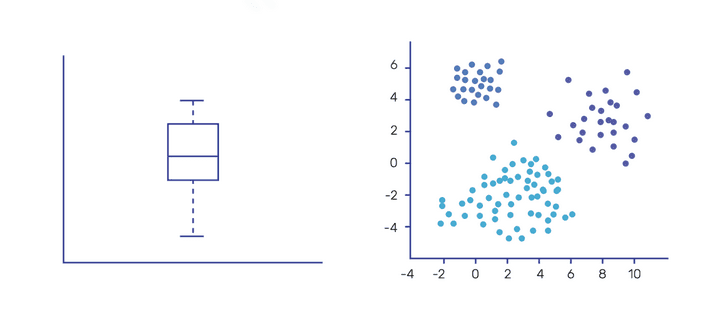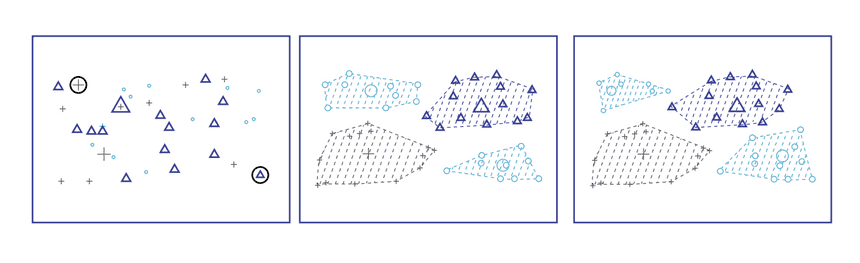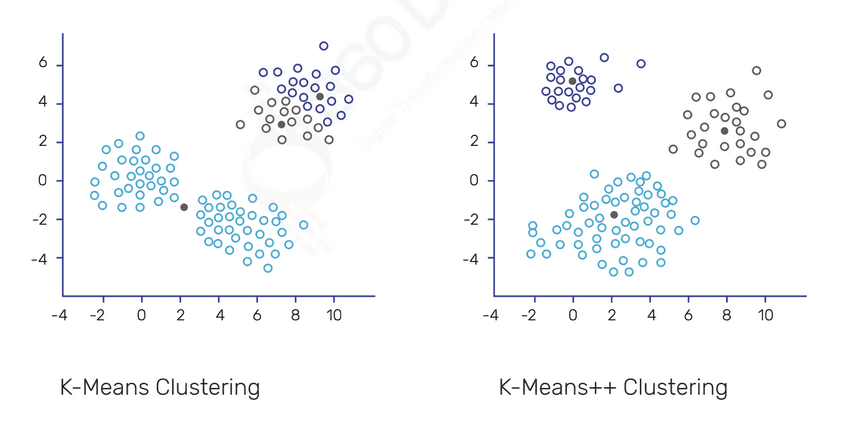Home / Blog / Data Science Digital Book / Types of Clustering / Segmentation Algorithms

# Types of Clustering / Segmentation Algorithms

• July 15, 2023
• 3266
• 21### Meet the Author : Mr. Bharani Kumar

Bharani Kumar Depuru is a well known IT personality from Hyderabad. He is the Founder and Director of Innodatatics Pvt Ltd and 360DigiTMG. Bharani Kumar is an IIT and ISB alumni with more than 17 years of experience, he held prominent positions in the IT elites like HSBC, ITC Infotech, Infosys, and Deloitte. He is a prevalent IT consultant specializing in Industrial Revolution 4.0 implementation, Data Analytics practice setup, Artificial Intelligence, Big Data Analytics, Industrial IoT, Business Intelligence and Business Management. Bharani Kumar is also the chief trainer at 360DigiTMG with more than Ten years of experience and has been making the IT transition journey easy for his students. 360DigiTMG is at the forefront of delivering quality education, thereby bridging the gap between academia and industry.

## Clustering / Segmentation

• Similar records to be grouped together. High intra-class similarity
• Dissimilar records to be assigned to different groups. Less inter-class similarity
• Heterogeneous groups will form homogeneous groups after clustering exercise
• Clustering is an exploratory technique
• Separation of clusters can be of two types:
Exclusive (one entry belongs to one cluster)
vs non-exclusive (one entry belongs to more than one cluster)

A straightforward boxplot may be used to do clustering when there is only one variable present. Scatter diagrams can be used when there are two variables.## Clustering / Segmentation

When we have more than 2 variables then there are a lot of other techniques such as:

### Partitioning Based Methods:

• K-Means Clustering
• K-Means++ Clustering
• Intelligence K-Means Clustering
• Genetic K-Means Clustering
• K-Medoids Clustering
• K-Medians Clustering
• K-Modes Clustering
• Weighted K-Means Clustering
• Kernel K-Means Clustering

## K-Means Clustering

Non-Hierarchical Clustering is the name given to K-Means clustering.

We use a Scree plot or an Elbow Curve to determine the number of clusters up front.

## Steps for K-Means

• Decide the number of clusters 'K' based on the elbow curve of scree plot or based on the thumb rule Sqrt(n/2). Alternatively, users may intuitively decide upon the number of clusters.
• Dataset is partitioned into ‘K’ clusters with 'K' centers called centroids. These centroids are randomly chosen as part of initialization.
• Each data point of the dataset, which is the closest to one of the centroids will form a cluster with that closest centroid
• Centroids are again recalculated with the data points assigned to each cluster
• Steps 3 & 4 are repeated iteratively until no further reassignment of data points to other clusters is possible.When centroids are initialised at random, the clustering exercise ends at a local minima (minima since the goal is to obtain the minimum inside the sum of squares).

The answer is to start the algorithm several times with various beginning partitions.

Although there are some general guidelines, they are not infallible. There is no established rule for choosing the K-value.

Solution: Run the algorithm with a variety of different 'K' values, and then choose the clusters with the lowest 'Within Sum of Square' and highest 'Between Sum of Square' values.

extremely sensitive to excessive values or outliers.

Solution: K-medians and K-Medoids are two more variations that effectively manage outliers.

When dealing with continuous data, K-Means clustering is effective.

Use K-Modes for categorical data as a solution.

Clusters with non-convex forms cannot be found.

Solution: Use Kernel K-Means and density-based clustering as a solution.

## K-Means++ Clustering

K-Means++ altogether addresses the problem of different initializations leading to different clusters.

### Steps:

• Decide the number of clusters 'K'
• First centroid is randomly selected
• Second centroid is selected such that, it is at the farthest end
• Step 2 depends on weighted probability score criteria
• This process continues until all 'K' centroids are obtained## K-Medians

K-Medians is excellent at coping with outliers.

The distance unit used is L1 Norm, often known as Manhattan Distance.

Steps and K-Means are quite similar, with the exception that we compute Median rather than Mean.

• K-Means cannot handle categorical data.
• Categorical data can be converted into one-hot encoding but will hamper the quality of the clusters, especially when the dimensions are large.
• K-Modes is the solution and uses modes instead of means and everything else is similar to K-Means.
• Distance is measured using frequency.
• If the data has a mixture of categorical and numerical data then the K-Prototype method can be used.
• K-Means can only handle linearly separable patterns and Kernel K-Means clustering works well when the data is in non-convex format (non-linearly separable patterns)
• Kernel functions are used to take data to high-dimensional space to make it linear and captures the patterns to form clusters.

## K-Medoids

K-Medoids address the problem of K-Means getting influenced by outliers.

### Steps:

• Choose 'K' data points randomly as medoids

• Instead of taking the centriod of data points of a cluster, medoids are considered to be the center.

• Find out the distance from each and every data point to the medoid and add them to get a value. This value is called total cost.

• Select any other point randomly as a representative point (any point other than medoid points)

• Find out the distance from each of the points to the new representative point and add them to get a value. This value is called the total cost of a new representative point.

• If the total cost of step 3 is greater than the total cost of step 5 then the representative point at step 4 will become a new medoid and the process continues.

• If the total cost of step 3 is less than the total cost of step 5 then the algorithm ends.

## Partitioning Around Medoids (PAM)

Partitioning Around Medoids (PAM) is a classic example of K-Medoids Algorithm.

### Steps:

• Randomly points are chosen to be medoids.
• Replace medoids will non-medoids, if the Total Cost (Sum of Squared Errors - SSE) of the resulting cluster is improved (reduced).
• Continue iteratively until the Total Cost criteria of step 2 is satisfied## CLARA - Clustering Large Applications:

• In the case of large datasets performing clustering by in-memory computation is not feasible. The sampling technique is used to avoid this problem

• CLARA is a variant of PAM.

• However unlike PAM, the medoids of all the data points aren’t calculated, but only for a small sample.

• The PAM algorithm is now applied to create optimal medoids for the sample.

• CLARA then performs the entire process for a specified no of points to reduce bias.

Click here to learn Data Science Course, Data Science Course in Hyderabad, Data Science Course in Bangalore

### Data Science Training Institutes in Other Locations